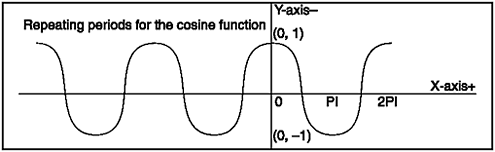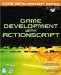# Periods, Domains, and Ranges

 [ LiB ]

All the functions discussed so far fall into the periodic function category. What this means is that sine and cosine will forever repeat their values after a period, no matter what value is fed to them. The horizontal interval that function values take to repeat is called the period of the function. This period is 2Pi for both sine and cosine.

NOTE

TIP

If you convert 2Pi to degrees you get 360 degrees.There goes that circle again. Try rearranging the radian formula so you can solve for degrees.

Figure 11.8 shows an example of an ongoing function period.

##### Figure 11.8. Repeating periodsFigure 11.8 shows what happens when you feed different values to a cosine curve. The curve was graphed for three periodsthat would be its domain. A function's domain is the starting point to the ending point of the graph. As I said, sine and cosine usually have 02PI for their domains. Sine and cosine domains are exactly one period long.

The range of a function is a bit different. The range of a function is the vertical limits of that function. This means that when you sketch out a sine wave, the vertical limits of the wave are usually 1 to 1. You can always scale these as I did in previous demos in this chapter.

 [ LiB ]Game Development with ActionScript
ISBN: 1592001106
EAN: 2147483647
Year: 2004
Pages: 162
Authors: Lewis Moronta